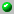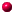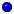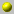Katsuhisa MIMACHI

The homepage can be accessed here.
Email : mimachi@math.kyushu-u.ac.jp

the following papers are available on our server= dvi format= ps format= tex format= pdf formata_new_derivation_of_the_inner_product_formula_for_the_macdonald_symmetric_polynomialsan_integral_representation_of_eigenfunctions_for_macdonalds_q_difference_operatorsan_integral_representation_of_eigenfunctions_for_macdonalds_q_difference_operatorsa_reproducing_kernel_for_nonsymmetric_macdonald_polynomialseigenfunctions_of_macdonalds_q_difference_operator_for_the_root_system_of_type_C_nmacdonalds_operator_from_the_center_of_the_quantized_universal_enveloping_algebra_U_q(gl(N))macdonalds_operator_from_the_center_of_the_quantized_universal_enveloping_algebra_U_q(gl(N))representations_of_the_hecke_algebra_on_a_family_of_rational_functionssingular_vectors_of_the_virasoro_algebra_in_terms_of_jack_symmetric_polynomials

Go back to the directory of pre and reprints.
Or search the database by keywords
Or look for the newest entires in the database